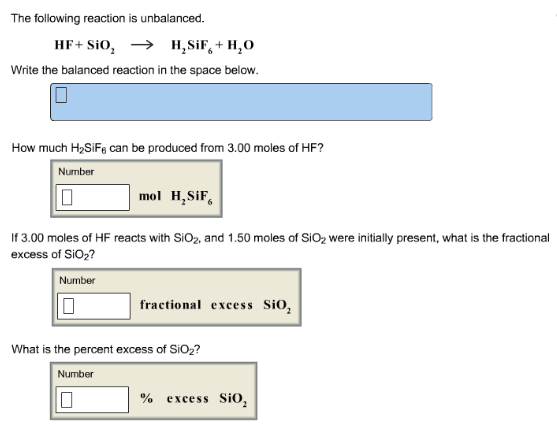# Problem: The following reaction is unbalanced. HF + SiO2 → H2SiF6 + H2O Write the balanced reaction in the space below. How much H2SiF6 can be produced from 3.00 moles of HF? If 3.00 moles of HF reacts with SiO2, and 1.50 moles of SiO2 were initially present, what is the fractional excess of SiO2? What is the percent excess of SiO2?

###### FREE Expert Solution
95% (169 ratings)###### Problem Details

The following reaction is unbalanced.

HF + SiO2 → H2SiF6 + H2

Write the balanced reaction in the space below.

How much H2SiF6 can be produced from 3.00 moles of HF?

If 3.00 moles of HF reacts with SiO2, and 1.50 moles of SiO2 were initially present, what is the fractional excess of SiO2

What is the percent excess of SiO2?